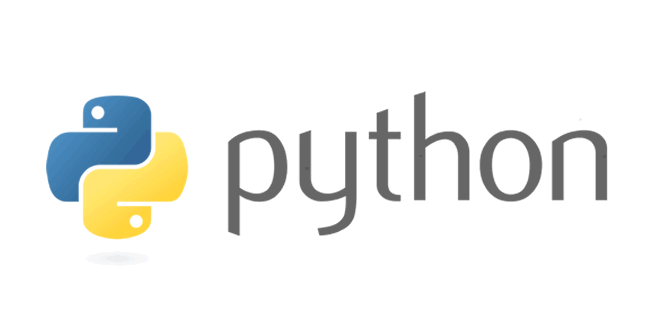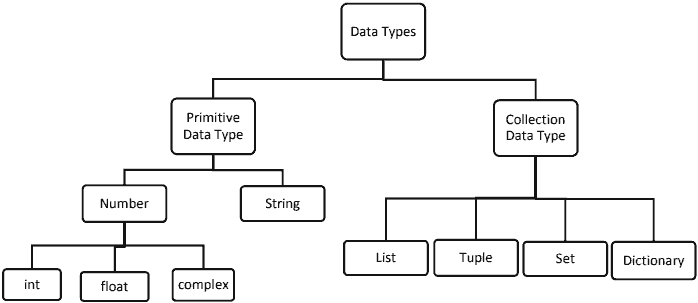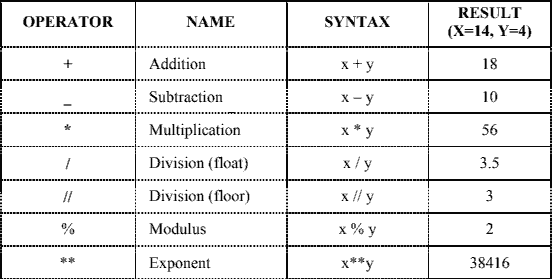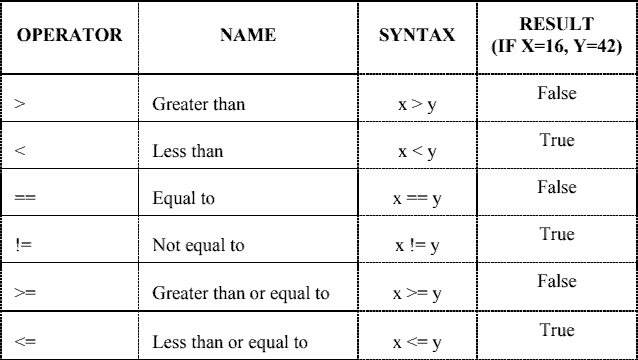Tuesday , August 9 2022# Data Handling: 11th Class Computer Science Chapter 03

 Chapter Name: Data Handling [Chapter 03] Class: 11th Subject: Computer Science

### 3.1 Data Types in Python: Ch 03 – Data Handling

Python has Two data types:

1. Primitive Data Type (Numbers, String)
2. Collection Data Type (List, Tuple, Set, Dictionary)### 1. Primitive Data Types:

#### a. Numbers: Number data types store numeric values.

There are three numeric types in Python:

• int
• float
• complex

Example:

 w = 1 # int y = 2.8 # float z = 1j # complex

#### integer: There are two types of integers in python:

• int
• Boolean

int: int or integer, is a whole number, positive or negative, without decimals.

Example:
x = 1
y = 35656222554887711
z = -3255522

Example:

>>> bool(0)
False
>>> bool(1)
True
>>> bool(‘ ‘)
False
>>> bool(-34)
True
>>> bool(34)
True

Example:

x = 1.10
y = 1.0
z = -35.59

a = 35e3
b = 12E4
c = -87.7e100

#### complex: Complex numbers are written with a “j” as the imaginary part.

Example:

>>>x = 3+5j
>>>y = 2+4j
>>>z=x+y
>>>print(z)
5+9j
>>>z.real
5.0
>>>z.imag
9.0

Real and imaginary part of a number can be accessed through the attributes real and imag.

#### b. String: Sequence of characters represented in the quotation marks.

• Python allows for either pairs of single or double quotes. Example: ‘hello’ is the same as “hello” .
• Python does not have a character data type, a single character is simply a string with a length of 1.
• The python string store Unicode characters.
• Each character in a string has its own index.
• String is immutable data type means it can never change its value in place

• List
• Tuple
• Set
• Dictionary

### 3.2 Mutable & Immutable Data Type: Ch 03 – Data Handling

• Mutable Data Type:
These are changeable. In the same memory address, new value can be stored.
Example: List, Set, Dictionary
• Immutable Data Type:
These are unchangeable. In the same memory address new value cannot be stored.
Example: integer, float, Boolean, string and tuple

### 3.3 Basic Operators in Python:

1. Arithmetic Operators
2. Relational Operator
3. Logical Operators
4. Bitwise operators
5. Assignment Operators
6. Other Special Operators
• Identity Operators
• Membership operators

#### i. Arithmetic Operators: To perform mathematical operations.Example:
>>>x= -5
>>>x**2
>>> -25

#### ii. Relational Operators: Relational operators compare the values. It either returns True or False according to the condition.#### iii. Logical operators: Logical operators perform Logical AND, Logical OR and Logical NOT operations.

 OPERATOR DESCRIPTION SYNTAX and Logical AND: True if both the operands are true x and y or Logical OR: True if either of the operands is true x or y not Logical NOT: True if operand is false not x

Examples of Logical Operator:

The and operator: The and operator works in two ways:

a. Relational expressions as operands
b. numbers or strings or lists as operands

a. Relational expressions as operands:

 X Y X and Y False False False False True False True False False True True True

>> 5 > 8 and 7 > 3
False
>>> (4==4) and (7==7)
True

b. numbers or strings or lists as operands:

In an expression X and Y, if first operand has false value, then return first operand X as a result, otherwise returns Y.

 X Y X and Y False False X False True X True False Y True True Y

0 and 0
0
>>> 0 and 6
0
>>> ‘a’ and ‘n’
’n’
>>> 6>9 and ‘c’+9>5 # and operator will test the second operand only if the first operand
False # is true, otherwise ignores it, even if the second operand is wrong

The or operator: The or operator works in two ways:

a. Relational expressions as operands
b. numbers or strings or lists as operands

a. Relational expressions as operands:

## 11th CBSE NCERT Computer Science Term 2 Question Paper 2021-22

School Name: Himalaya Public School, Sector 13, Rohini, Delhi 110085 India Class: 11th Standard (CBSE) Subject: Computer Science …